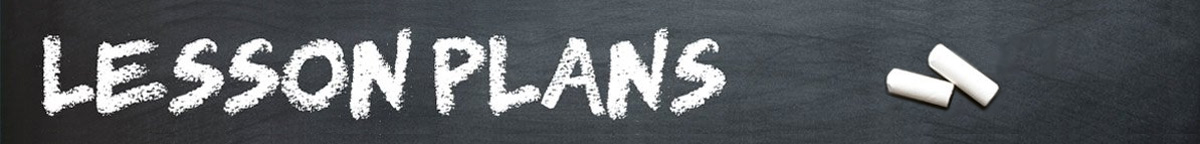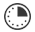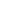# Silent Geometry

JRDAssist
Middle School and High School Teacher

The students will practice using geometry formulas measuring items in the classroom to find area and volume, radius and circumference, and identify the types of angles.

##Length of Time: About 1 - 2 Class Periods

Featured Programs:

### Common Core Alignment

CCSS.Math.Content.7.G.B.4 - Know the formulas for the area and circumference of a circle and use them to solve problems; give an informal derivation of the relationship between the circumference and area of a circle.

CCSS.Math.Content.7.G.B.5 - Use facts about supplementary, complementary, vertical, and adjacent angles in a multi-step problem to write and solve simple equations for an unknown angle in a figure.

CCSS.Math.Content.7.G.B.6 - Solve real-world and mathematical problems involving area, volume and surface area of two- and three-dimensional objects composed of triangles, quadrilaterals, polygons, cubes, and right prisms.

## Objectives & Outcomes

The students will be able to correctly use area and volume formulas for various figures, circles, and polygons, and identify angle types and the degrees of angles.

## Materials Needed

• calculator
• protractor
• ruler (yardstick, measuring tape)
• measuring chart to record information

Prepare ahead of time:  For each student-Measuring Chart with columns to list: name of item, shape or figure, dimension columns (h, l, w, d, etc.), perimeter, area, volume, angle types

## Procedure

### Opening to Lesson

• Once students are seated the teacher will ask for a volunteer to the front of the room
• Using a tape measure the teacher will measure the height of the student
• Teacher will ask students: What does that number (height) mean?
• Accept all logical responses
• Tell students that everything has area or volume or size, if it can be seen (even unseen) it can be measured

### Body of Lesson

#### Modeling

• Distribute the measuring chart to each student
• Display an example from the measuring chart with each column shown
• Give the tape measure for the volunteer to measure the door, the height, width, and depth (or thickness)
• Display the measurements
• Ask students what could be found using those dimensions (volume, area, surface area)
• Have students use their calculators to find volume, area, etc.

#### Guided Practice

• Explain the rules to the students: This is silent geometry, no talking or sharing answers
• Clarify the instructions on the Measurement Chart, every possible item in the room can be measured to figure the area, volume, angle type, etc.
• Students are to identify the item, the measurements, etc., using the columns on the Measurement Chart
• Distribute supplies
• Allow students to begin roaming the classroom to measure as many items as possible in 30 minutes
• Encourage students to measure all items regardless of its size
• Students are to figure the area, volume, etc. before moving onto another item
• Students may estimate some of the irregular items
• After 30 minutes students return to their seats to complete final calculations
• Stress that the charts should be neat and organized
• Collect all work

#### Independent Practice

• Homework assignment: Students do the same exercise at home measuring a set number of items throughout their bedroom or other room in the home.

### Closing

Ask students:  What is the purpose of measuring things in the world?  Discuss all of the purposes for knowing the sizes, volumes, etc. of things whether in nature or other places.

## Assessment & Evaluation

Teacher-created or commercial worksheet with word problems related to geometric formulas given as homework or an in-class quiz, test, or assignment

## Modification & Differentiation

Students may work in pairs.  Allow students to talk quietly but cannot share answers with other students.  Hold the class outdoors.  Limit number of items to measure.  Increase or decrease the time limit.  Have students do the calculations once all measurements are made at their seats.  Give a list of premeasured items and students must match it to an item in the classroom and calculate its area, volume, etc.

## Related Lesson Plans

##### Interest-ing Math

Students will learn about simple interest and how to calculate the real cost of a loan, credit card, and other types of borrowing.

##### Order of Operations

This lesson is designed to help students solve problems involving the four operations, and identify and explain patterns in arithmetic.

##### Order in the Math Class

The students will physically review the order of operations.

##### Prices and Percentages

Using a current list of prices for food and clothing, the students will practice math skills related to percentages.

##